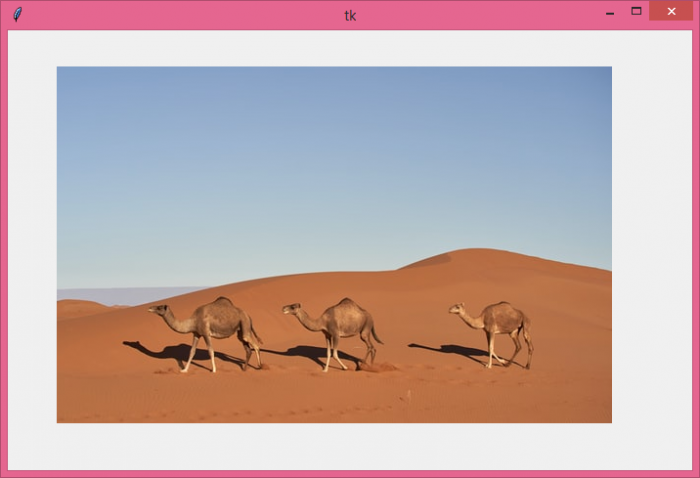# Embedding an Image in a Tkinter Canvas widget using PIL

The Pillow library in Python contains all the basic image processing functionality. It is an open-source library available in Python that adds support to load, process, and manipulate the images of different formats.

Let's take a simple example and see how to embed an Image in a Tkinter canvas using Pillow package (PIL). Follow the steps given below −

## Steps −

• Import the required libraries and create an instance of tkinter frame.
from tkinter import *
from PIL import Image, ImageTk
• Set the size of the frame using root.geometry method.

• Next, create a Canvas widget using canvas() function and set its height and width.

• Open an image using Image.open() and then convert it to an PIL image using ImageTk.PhotoImage(). Save the PIL image in a variable "img".

• Next, add the PIL image to the Canvas using canvas.create_image().

• Finally, run the mainloop of the application window.

## Example

# Import the required Libraries
from tkinter import *
from PIL import Image, ImageTk

# Create an instance of tkinter frame
root = Tk()

# Set the geometry of tkinter frame
root.geometry("700x450")

# Create a canvas widget
canvas= Canvas(root, width=600, height=400)
canvas.pack()

img=ImageTk.PhotoImage(Image.open("camels.jpg"))

# Add image to the Canvas Items
canvas.create_image(250, 250, anchor=CENTER, image=img)

root.mainloop()

## Output

When you run this code, it will produce the following output window −